This blog has moved ! New look and new articles on www.naturelovesmath.com/en/

# What is time ?

 Easy : for the curious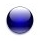Sundial of Saint Rémy de Provence Source : Wikipedia Commons

« Put your hand on a stove for a minute and it will feel like an hour. Sit with a pretty girl for an hour and it will fly like a minute. That's relativity. »

This famous quote, attributed to Einstein 1 is a good description of the psychological side of time. Time is undeniably linked to our senses, we perceive it through duration, order and simultaneity. What will be left of it if we take those away ?

The physicist and/or mathematician is used to depicting time rather than asking himself about its nature. For him, time is more of a variable, it's the degree of freedom of bodies that interact or move in space. Concretely, this parameter permits us to measure durations (chronometry), and mark order (chronology). We can mathematically define these properties : duration is the locus in a one-dimensional space, order is represented by the orientation of this straight line.

We will see that this mathematical representation of time as a supplementary dimension of space is not characteristic of relativity theory, and Galilean kinematics can use it as well. We will then see clearly the fundamental differences between the concept of time in the two theories and we will try to understand the geometry behind the notorious change of sign of the mathematician's "ds²". We will also see that during the evolution of physical science, time has progressively lost what we thought were fundamental properties of his...

## The "absolute" or global time

Inherited from Newton's mechanics, the "absolute" time, may it exists or be an a priori of consciousness, is homogeneous and flows separated from phenomenon and in particular from observers. Note that the term "absolute" belongs to philosophy's linguistics, and doesn't make much sense in physics, hence the inverted commas.

For the mathematician, homogeneity implies that space-time can be pictured as an affine (four-dimensional) space : each point of space-time (called an event) is exactly the same and can be sent on another one using a translation.
The fact that time is separate from observers allows us to define simultaneity. Two distinct events are said simultaneous if they happen "at the same time", meaning they have the same time coordinate value :All the points on the orange line have the same time coordinate value for every observer

So in classical mechanics time is just a dimension amongst the other, its only characteristic being its orientation 2. Space-time is cut in slices of space, literally.Classical three-dimensional space-time is cut in slices

In mathematics, one says that it is a fiber bundle (a trivial one at that). At each instant (element of the basis of the bundle, the time dimension) is associated a fiber of space independent of others. Each fiber is like a "set of simultaneous events".
In two-dimensional space-time, fibers are straight lines of simultaneity. In three dimensions, those are planes of simultaneity, and in four-dimensional space-time, each fiber is a three-dimensional space of simultaneity.
In clear, space-time is like a "flip book" whose pages are one, two or three-dimensional respectively.

Here is an example of a flip book, metaphorically picturing a three dimensional (trivial) fiber bundle, each page being one fiber :

 Each page of the flip book is a fiber, or two-dimensional simultaneity space Source : Youtube - probable viral video for the advertising of the LG viewty

In this classical space-time, there is no limitation of speed : if two simultaneous events are linked by a "messenger", he moves at infinite speed.
The geometric behavior of events is said to be euclidean : the distance between two events
$X=(x, y, z, t)$ and $X^\prime=(x^\prime, y^\prime, z^\prime, t^\prime)$
is
$d(X,X^\prime)^2=(x-x^\prime)^2+(y-y^\prime)^2+(z-z^\prime)^2+(t-t^\prime)^2$.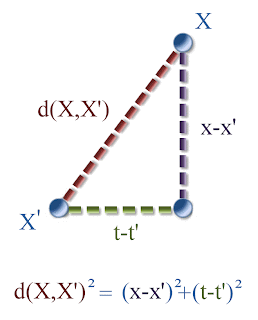Euclidean distance between two points in a plane

Surely you recognized... Pythagoras !« In any right triangle, the area of the square whose side is the hypotenuse is equal to the sum of the areas of the squares whose sides are the two legs »

What is this famous theorem doing in this matter ?
It permits us to calculate the coordinates of points with respect to the geometry. Let's take all the points at a unitary distance of the origin. Those are the points $X$ with coordinates $(x,y)$ so that $d(X,O)=x^2+y^2=1$, which is the equation of a circle, and it's precisely what characterizes euclidean geometry !Euclidean unitary distance given by Pythagoras' theorem

## The "relative" or local time

What's new with relativity theory is that the concept of time becomes local. Space-time is not a fiber bundle and simultaneity doesn't make sense anymore, because it depends on the observer's reference frame. Each observer has a different affine frame, depending on its speed relatively to another frame of reference.

For example, let's take an inertial reference frame in a two-dimensional space-time. It consists in considering an oriented affine frame with a metric in the plane (see the article about the group of special relativity for more details on affine reference frames). In this frame all simultaneous events, so all points on a horizontal straight line (if we chose to represent time on the ordinate) - in the coming example it would be the green line - are simultaneous only for an observer measuring spatiotemporal distances in this particular frame, that is to say at rest relatively to this reference frame.Line of simultaneous events in a reference frame

So, to observe events from a uniform rectilinear moving frame relatively to the latter is to change the affine frame. One changes it with a rotation 3 of the axis through an angle $\phi$. Note that the distance must be preserved, and it is not defined by a circle anymore but by a hyperbola in the plane (a three-dimensional hyperboloid in four-dimensional space). So the points on the hyperbola must slide along while the axis are rotating :Two affine frames => Two different lines of simultaneity

By putting the second affine frame over the other, we can see a new different line of simultaneity (in purple). It means that the events that were simultaneous when seen at rest, no longer are when moving, and other events are seen simultaneous.
To each event corresponds an infinity of spaces of simultaneity, depending upon its universe line (its direction and speed).

In this relativistic space-time, there exists a speed limit that no messenger can go beyond. We represent this by a cone in each affine frame : an observer coming from the origin can only move in a direction comprised in the cone (and only in its upper part to respect causality) :The light cone limits the speed of movement

The geometry of this space-time is called hyperbolic : the distance between two events
$X=(x, y, z, t)$ and $X^\prime=(x^\prime, y^\prime, z^\prime, t^\prime)$
is
$d(X,X^\prime)^2=(x-x^\prime)^2+(y-y^\prime)^2+(z-z^\prime)^2-(t-t^\prime)^2$.

That simple change of sign in the metric's definition or « ds² » to those who already know it, corresponds to the fact that a rotation of the axis no longer respects a unitary sphere as in euclidean geometry, but a unitary hyperboloid. It is not the formula of Pythagoras that will be used but another: this time, in two dimension, the points $X$ with coordinates $(x,y)$ at distance 1 from the origin verify $x^2-t^2=1$. These are not the couples of coordinates that determine the sides of a right-angled triangle with an hypotenuse of length 1, but rather those whose square have a difference of 1. Since the squares always have a difference of one, it means that the points of unitary distance from the origin move closer and closer to the light cone (of equation $x^2-t^2=0$) without ever touching it.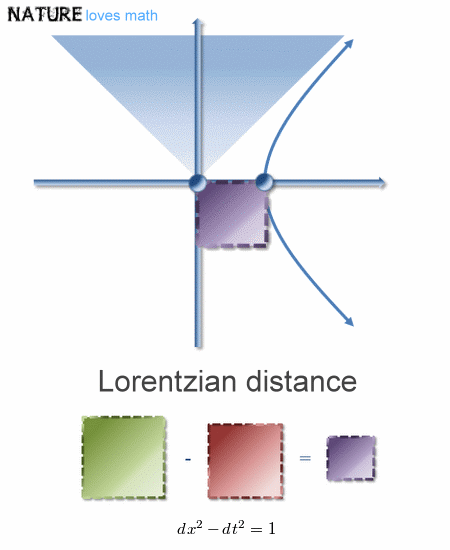Unitary Lorentzian "Distance" : $x^2$ (area of the green square) minus $t^2$ (area of the red square) equals $1$ (area of the purple square)

The same reasoning applies to the events located in the light cone, with just reversing the roles. 4 : the "distance" is $t^2-x^2$. We then have four branches of hyperbola to measure "distances" of events from the origin, in or out of the cone, in the past or future of the origin event :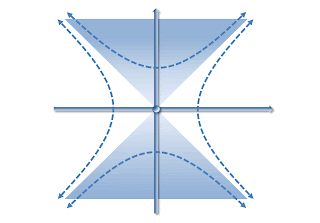The four branches of the hyperbola in two dimension

Note that the generalization to three and four dimensions poses no problem whatsoever : the hyperbola becomes a hyperboloid surface, and a hyperboloid space respectively (that one is harder to picture). The metric that links squares areas in two dimensions just links more squares in superior dimensions (and not cubes or hypercubes volumes as we would like to think intuitively).
As for the constant $c$ in the metric that we deliberately omitted for the sake of simplicity, it only means the cone is not right-angled but squeezed...

And what does all this mean physically ? It means that the measure of time, the chronometry, depends upon the path of the observer !

If two observers move from the origin of space-time (so from the same point in space and at the same time, provided they are both at rest first), and go eparate ways before joining again in the future, which path would be the shorter ? the "faster" ?The blue observer stays at rest while the red one makes a fast round-trip

The points in some affine frame have the coordinates :
$A=(0,0)$, $B=(x,t^\prime)$, $C=(0,t)$.

The distance $d(A,C)=t$ is that traveled in space-time by the observer at rest.

We have $d(A,B)=\sqrt{t^{\prime 2}-x^2} \leq t^\prime$

and $d(B,C)=\sqrt{(t-t^\prime)^2-x^2} \leq t-t^\prime$.

So that $d(A,B)+d(B,C)\leq t^\prime+(t-t^\prime)=t=d(A,C)$.

In conclusion the moving observer has taken the shortest path in space-time ! The shortest path between two points is not the straight line anymore, we have a non-euclidean geometry.

Note that to simplify calculations, we did not take into account any acceleration of the moving observer, its trajectories are then straight lines. He moves first at a null speed (when he's at rest with the other observer), then suddenly at a non-zero (constant) speed, then suddenly at the same speed but in the opposite (spatial) direction, then again at zero speed, without any transition... This behavior certainly isn't very realistic.
To take accelerations into account, one would just need to consider curved lines, along which each light cone would be tilted depending upon the velocity vector. We would then see that the observers are not in a symmetrical position, the acceleration having physical consequences distinguishing the accelerated frame from the inertial one. No need to lost ourselves into the generalized theory of relativity, contrary to popular beliefs.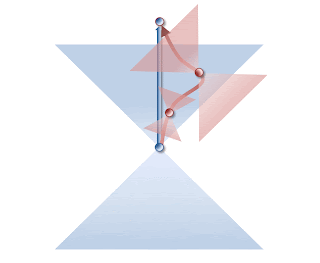Diagram taking account of accelerations

## Conclusion

So there is the meaning of the sign change in the metric : space-time loses its fiber bundle structure for a more complex one. Each fiber is replaced with an infinity of simultaneity spaces depending on the observer...
Time is not "absolute" anymore, it is observer-dependent. Each observer has its "proper time", that's why we say time has become local.

And what about other theories ? In general relativity time (as well as space) also loses its homogeneity : we do not represent space-time with a flat affine space, but with a more general concept of space called a "manifold". It is a curved space-time, and it is not possible to send an event to another by means of a simple translation anymore.
In quantum mechanics, there is no preferred dimension to be called time. Time is not a physical variable anymore, and has become an arbitrary-chosen independent variable.
In the recent theories, draftings of quantum gravity, it... doesn't even exist anymore ! How could that be ?

Let's put aside the mathematical representations of time, and try to answer the question of the nature of durations (rather than that of time).
What are we talking about when we says we're 45 years old ? We are stating that between the moment we were born and now, our planet earth revolved 45 times around the sun.
And when we say that an athlete only needs 9.58 seconds to run 100 meters ? It means that between start and finish, there has been exactly $9,58 \times 9\, 192\, 631\, 770 = 88\, 065\, 412\, 360$ changes of state of the cesium 133 atom at rest.

In other words, we never measure time itself. Any measure of duration is only a comparison between several physical variables. That's why we do not know anything about "physical time" : it doesn't exist ! The variable $t$ is just a useful tool to compare physical variables that have some regularity.
Indeed, according to Carlo Rovelli, « the idea of a time "t" that itself flows, and along which everything evolves, is not a fruitful idea anymore. The world cannot be described by equations of evolution in a time "t". [...] Instead of measuring everything within the "time", abstract and absolute, which was a "trick" invented by Newton, we can describe each variable according to the state of other variables. » 5 (C.Rovelli What is time, what is space?, Di Renzo Editore, 2006)

#### Notes :

1. It is a paraphrase of the original quote : « When a man sits with a pretty girl for an hour, it seems like a minute. But let him sit on a hot stove for a minute and it's longer than any hour. That's relativity ».
Personal statement from A.Einstein to his secretary Helen Dukas. Cited in Contemporary quotations, James Beasley Simpson, p156, 1957. Also in Expandable Quotable Einstein, edited by Alice Calaprice, 2005.

2. Space also is oriented but in a three-dimensional way, with an orientation of rotations. The time orientation is one-dimensional, it is a direction. see the article about the group of special relativity for more details on affine frames in special relativity

3. The angle $\phi$ of this rotation is so that $\tan\phi=\dfrac vc$ with $v$ the speed of the new frame relatively to the other and $c$ the speed of light in vacuum. Strictly speaking, it is not really an ordinary rotation, but a hyperbolic one, explaining the fact that both axis have to be rotated in both directions, to "respect the hyperbola".

4. It is because the quadratic form is not positive-definite anymore, and two cases present themselves to define a distance, which must by definition always be positive.

5. « l'idée d'un temps "t" qui s'écoule de lui-même, et par rapport auquel tout le reste évolue, n'est plus une idée efficace. Le monde ne peut pas être décrit par des équations d'évolution dans le temps "t". [...] Plutôt que de tout rapporter au "temps", abstrait et absolu, ce qui était un "truc" inventé par Newton, on peut décrire chaque variable en fonction de l'état des autres variables.» (C.Rovelli Qu'est-ce que le temps ? Qu'est-ce que l'espace ?, Bernard Gilson Editeur, 2008, p83)0 Responses to "What is time ?"